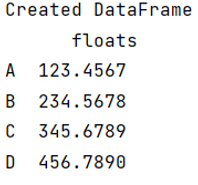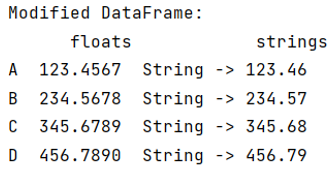# How to display Pandas DataFrame of floats using a format string for columns?

Here, we have to display Pandas DataFrame of floats using a format string for columns. By Pranit Sharma Last updated : September 21, 2023

Pandas is a special tool which allows us to perform complex manipulations of data effectively and efficiently. Inside pandas, we mostly deal with a dataset in the form of DataFrame. DataFrames are 2-dimensional data structure in pandas. DataFrames consists of rows, columns and the data.

## Problem statement

Given a Pandas Dataframe with floats, we have to display these values using a format string for columns.

## Displaying Pandas DataFrame of floats using a format string for columns

Pandas offers us a feature to convert floats into a format of string. This can be done in multiple ways but here we are going to use map() method.

Let us understand with the help of an example,

## Create a dataframe with float values

```# Importing pandas package
import pandas as pd

# Creating a dictionary
d = {'floats':[123.4567, 234.5678, 345.6789, 456.7890]}

# Creating a DataFrame
df = pd.DataFrame(d,index=['A','B','C','D'])

# Display created DataFrame
print("Created DataFrame\n",df)
```

### Output## Convert/Display floats using a format string for columns

Here, since all the values of the column floats having the data type float, we are now going to use map() method to convert all the floats into string.

```# Using map method to convert all the floats into string
# Also all the converted values will go into
# another column called strings
df['strings'] = df['floats'].map('String -> {:,.2f} '.format)

# Display modified DataFrame
print("Modified DataFrame:\n",df)
```

### Output